# 1753 What Is the Slope of the Driveway?

### Today’s Puzzle:

With so much snow all around it is easy to think about summer. Last summer my family and I stayed at a magnificent Airbnb. There was more than enough room for my husband and me, our children and their spouses, and our grandchildren (32 people in total).

The owners of the Airbnb wrote that the best views come from homes with steep driveways. I will share some of those views later in the post. But just how steep was the driveway? I took a picture and added a grid so you can calculate the slope. Notice that the driveway zigzags. You can estimate where the front tire of the van parked at the top of the driveway hits the pavement to calculate the slope of the top part of the driveway.Here’s the top half of the driveway:

###The beautiful view from the top of the driveway:

###The next three pictures are gorgeous views from inside the house or from a balcony:

###The views definitely made the steep driveway worth it!

Since this is my 1753rd post, I’ll write a little about that number.

### Factors of 1753:

• 1753 is a prime number.
• Prime factorization: 1753 is prime.
• 1753 has no exponents greater than 1 in its prime factorization, so √1753 cannot be simplified.
• The exponent in the prime factorization is 1. Adding one to that exponent we get (1 + 1) = 2. Therefore 1753 has exactly 2 factors.
• The factors of 1753 are outlined with their factor pair partners in the graphic below.How do we know that 1753 is a prime number? If 1753 were not a prime number, then it would be divisible by at least one prime number less than or equal to √1753. Since 1753 cannot be divided evenly by 2, 3, 5, 7, 11, 13, 17, 19, 23, 29, 31, 37, or 41, we know that 1753 is a prime number.

### More About the Number 1753:

1753 is a powerful sum:
2¹º + 3⁶ = 1753.

Since both of those exponents are even, 1753 is also the sum of two squares:
32² + 27² = 1753.

1753 is the hypotenuse of a Pythagorean triple:
295-1728-1753, calculated from 32² – 27², 2(32)(27), 32² + 27².

Here’s another way we know that 1753 is a prime number: Since its last two digits divided by 4 leave a remainder of 1, and 32² + 27² = 1753 with 32 and 27 having no common prime factors, 1753 will be prime unless it is divisible by a prime number Pythagorean triple hypotenuse less than or equal to √1753. Since 1753 is not divisible by 5, 13, 17, 29, 37, or 41, we know that 1753 is a prime number.

# 1747 Getting a Super Bowl Ring Isn’t Easy

### Today’s Puzzle:

On Wednesday I read an article in my local paper titled From undrafted free agent to the biggest stage: Britain Covey’s ‘unique ride’ to Super Bowl LVII. It got me quite excited for today’s game. Unfortunately, between Wednesday and Sunday, Covey suffered a hamstring injury.  Getting a Super Bowl Ring certainly isn’t easy. Being able to contribute to your team’s winning the game isn’t easy either. UPDATE: Good News! He was able to make at least one play in the second quarter! Further update: Even though his team lost the game in the final minutes, this rookie played well when he was on the field. I think both teams played exceptionally well and gave us all an exciting game to watch.

Before the game, it is a mystery which team will win the game. The difficulty level of this puzzle is a mystery also.

If you look at today’s puzzle just right, I think it looks a little like a super bowl ring, but I forwarn you, it will not be easy to get this puzzle either. You will need to place the numbers from 1 to 12 both in the first column and in the top row so that the given clues are the products of the numbers you place. Logic and practice will get you there. Good Luck!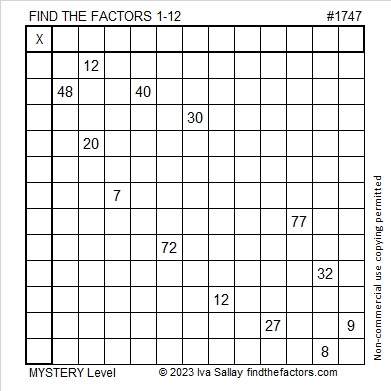You also might enjoy this next Super Bowl puzzle I saw on Twitter:

### Factors of 1747:

• 1747 is a prime number.
• Prime factorization: 1747 is prime.
• 1747 has no exponents greater than 1 in its prime factorization, so √1747 cannot be simplified.
• The exponent in the prime factorization is 1. Adding one to that exponent we get (1 + 1) = 2. Therefore 1747 has exactly 2 factors.
• The factors of 1747 are outlined with their factor pair partners in the graphic below.How do we know that 1747 is a prime number? If 1747 were not a prime number, then it would be divisible by at least one prime number less than or equal to √1747. Since 1747 cannot be divided evenly by 2, 3, 5, 7, 11, 13, 17, 19, 23, 29, 31, 37, or 41, we know that 1747 is a prime number.

### More About the Number 1747:

1747 is a palindrome in base 15.
It’s 7B7₁₅ because 7(15²) + 11(15) + 7(1) = 1747.

# 1741 What Do You Notice About the Remainders?

### Today’s Puzzle:

The table below is a list of the first 280 prime numbers. Below each prime number is its remainder when the prime number is divided by 6. What do you notice about the remainders? What do you wonder?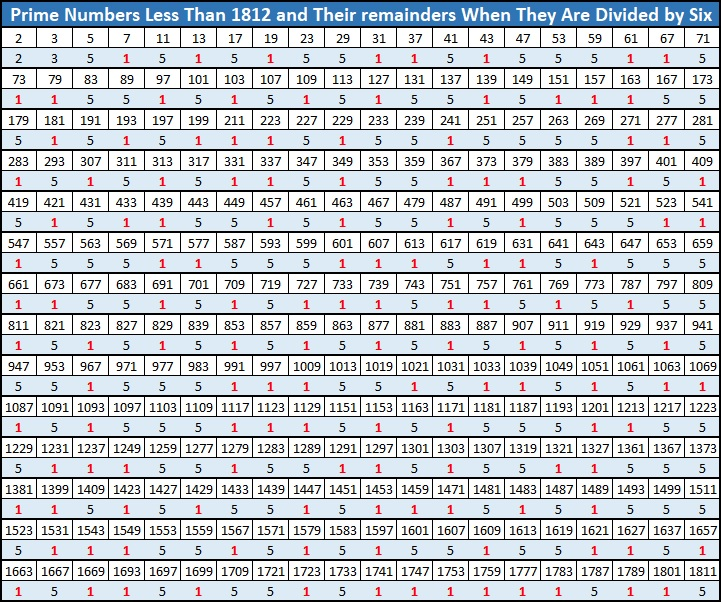Did you notice a fun fact starting with the number 1741? I would like to thank OEIS.org for making me aware of it.

### Factors of 1741:

• 1741 is a prime number.
• Prime factorization: 1741 is prime.
• 1741 has no exponents greater than 1 in its prime factorization, so √1741 cannot be simplified.
• The exponent in the prime factorization is 1. Adding one to that exponent we get (1 + 1) = 2. Therefore 1741 has exactly 2 factors.
• The factors of 1741 are outlined with their factor pair partners in the graphic below.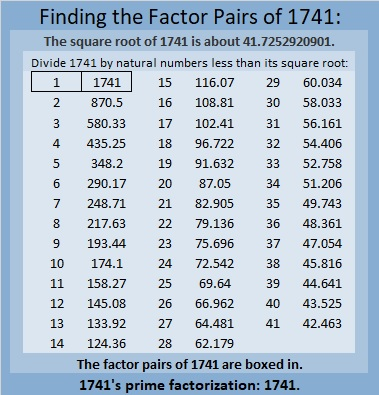How do we know that 1741 is a prime number? If 1741 were not a prime number, then it would be divisible by at least one prime number less than or equal to √1741. Since 1741 cannot be divided evenly by 2, 3, 5, 7, 11, 13, 17, 19, 23, 29, 31, 37, or 41, we know that 1741 is a prime number.

### More About the Number 1741:

1741 is the sum of two squares:
30² + 29² = 1741.

Here’s another way we know that 1741 is a prime number: Since its last two digits divided by 4 leave a remainder of 1, and 30² + 29² = 1741 with 30 and 29 having no common prime factors, 1741 will be prime unless it is divisible by a prime number Pythagorean triple hypotenuse less than or equal to √1741. Since 1741 is not divisible by 5, 13, 17, 29, 37, or 41, we know that 1741 is a prime number.

Since 29 and 30 are consecutive numbers, we have another fun fact: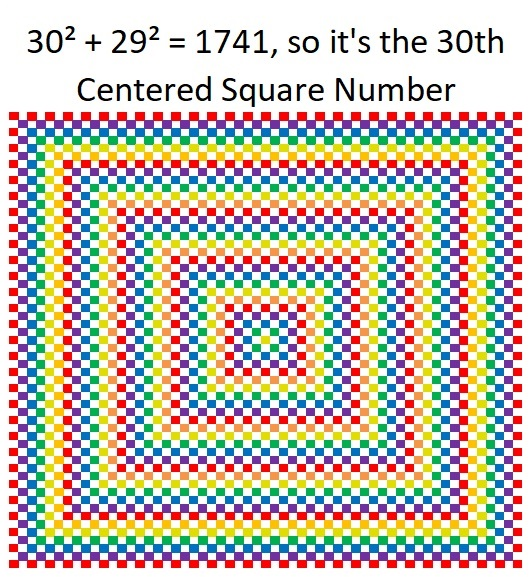And 1741 is the hypotenuse of a Pythagorean triple:
59-1740-1741, calculated from 30² – 29², 2(30)(29), 30² + 29².

1741 is also the difference of two consecutive squares:
871² – 870² = 1741.

# 1723 A Little Virgács

### Today’s Puzzle:

I haven’t blogged much this year so I guess I deserved a little bit of virgács in my shoes this morning. Mikulás (St. Nick) leaves virgács in the boots of naughty little boys or girls in the wee hours of December 6. Treats are for the good kids. What is virgács? It is small golden spray-painted twigs bound with some pretty red ribbon.  Of course, all children are sometimes naughty and sometimes nice, so they could all expect to get virgács along with their treats in their boots this morning.

You can solve this virgács puzzle by starting with the clues at the top of the grid, finding their factors, and working down the puzzle row by row until you have found all the factors. Each number from 1 to 10 must appear exactly one time in both the first column and the top row.### Factors of 1723:

• 1723 is a prime number.
• Prime factorization: 1723 is prime.
• 1723 has no exponents greater than 1 in its prime factorization, so √1723 cannot be simplified.
• The exponent in the prime factorization is 1. Adding one to that exponent we get (1 + 1) = 2. Therefore 1723 has exactly 2 factors.
• The factors of 1723 are outlined with their factor pair partners in the graphic below.How do we know that 1723 is a prime number? If 1723 were not a prime number, then it would be divisible by at least one prime number less than or equal to √1723. Since 1723 cannot be divided evenly by 2, 3, 5, 7, 11, 13, 17, 19, 23, 29, 31, 37, or 41, we know that 1723 is a prime number.

### More About the Number 1723:

1723 and 1721 are twin primes.

1723 is the sum of consecutive numbers:
861 + 862 = 1723.

1723 is the difference of consecutive squares:
862² – 861² = 1723.

# 1721 A Gift With Multiple Treasures

### Today’s Puzzle:

‘Tis the season of giving, and here’s a gift with multiple treasures inside. Write the numbers from 1 to 10 in both the first column and the top row so that the given clues are the products of the numbers you write. After you find the factors, you can complete the puzzle by finding all of the products.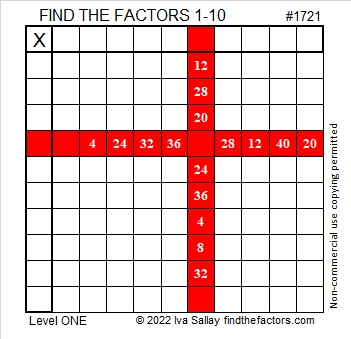### Factors of 1721:

• 1721 is a prime number.
• Prime factorization: 1721 is prime.
• 1721 has no exponents greater than 1 in its prime factorization, so √1721 cannot be simplified.
• The exponent in the prime factorization is 1. Adding one to that exponent we get (1 + 1) = 2. Therefore 1721 has exactly 2 factors.
• The factors of 1721 are outlined with their factor pair partners in the graphic below.How do we know that 1721 is a prime number? If 1721 were not a prime number, then it would be divisible by at least one prime number less than or equal to √1721. Since 1721 cannot be divided evenly by 2, 3, 5, 7, 11, 13, 17, 19, 23, 29, 31, 37, or 41, we know that 1721 is a prime number.

### More About the Number 1721:

1721 is the sum of two squares:
40² + 11² = 1721.

Here’s another way we know that 1721 is a prime number: Since its last two digits divided by 4 leave a remainder of 1, and 40² + 11² = 1721 with 40 and 11 having no common prime factors, 1721 will be prime unless it is divisible by a prime number Pythagorean triple hypotenuse less than or equal to √1721. Since 1721 is not divisible by 5, 13, 17, 29, 37, or 41, we know that 1721 is a prime number.

1721 and 1723 are twin primes.

# 1709 Sometimes “Guess and Check” Is a Good Strategy

### Today’s Puzzle:

Most of the puzzles I publish are logic puzzles, and I encourage you to find the logic of the puzzle and not guess and check.  However, guess and check is a legitimate strategy in mathematics, and it is a legitimate strategy to solve this particular puzzle.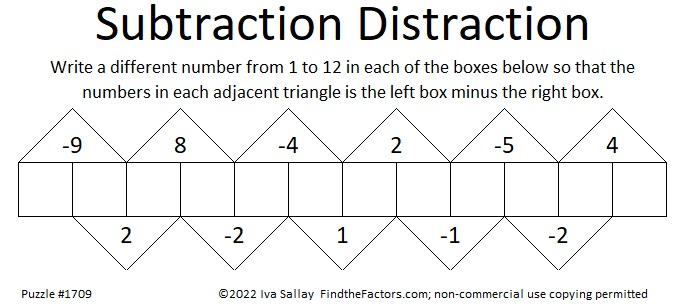Since one of the clues is -9, we know that the two boxes under it must be [1, 10], [2, 11], or [3, 12].

Suppose you assume it’s 1 – 10 = -9. If you fill out the rest of the boxes you would get: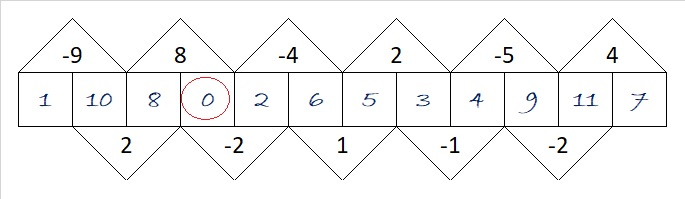You know that isn’t right because zero is not a number from 1 to 12. No problem. Simply add one to each of the numbers you wrote in, and the puzzle will be solved with only numbers from 1 to 12.

Suppose you assumed it’s 3 -12 = -9. The rest of the boxes would look like this:Again, 13 is not included in the numbers from 1 to 12, but you can fix it by subtracting 1 from each of the numbers you wrote in. Easy Peasy.

### Factors of 1709:

• 1709 is a prime number.
• Prime factorization: 1709 is prime.
• 1709 has no exponents greater than 1 in its prime factorization, so √1709 cannot be simplified.
• The exponent in the prime factorization is 1. Adding one to that exponent we get (1 + 1) = 2. Therefore 1709 has exactly 2 factors.
• The factors of 1709 are outlined with their factor pair partners in the graphic below.How do we know that 1709 is a prime number? If 1709 were not a prime number, then it would be divisible by at least one prime number less than or equal to √1709. Since 1709 cannot be divided evenly by 2, 3, 5, 7, 11, 13, 17, 19, 23, 29, 31, 37, or 41, we know that 1709 is a prime number.

### More About the Number 1709:

1709 is the sum of two squares:
35² + 22² = 1709.

1709 is the hypotenuse of a Pythagorean triple:
741-1540-1709, calculated from 35² – 22², 2(35)(22), 35² + 22².

Here’s another way we know that 1709 is a prime number: Since its last two digits divided by 4 leave a remainder of 1, and 35² + 22² = 1709 with 35 and 22 having no common prime factors, 1709 will be prime unless it is divisible by a prime number Pythagorean triple hypotenuse less than or equal to √1709. Since 1709 is not divisible by 5, 13, 17, 29, 37, or 41, we know that 1709 is a prime number.

# 1699 Sweet Candy Cane

### Today’s Puzzle:

Solving this candy cane puzzle can be a sweet experience. Just use logic to write the numbers 1 to 12 in both the first column and the top row so that those numbers and the given clues make a multiplication table.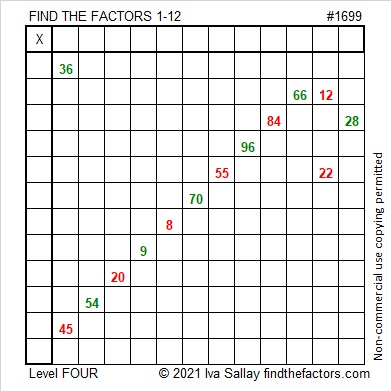### Factors of 1699:

• 1699 is a prime number.
• Prime factorization: 1699 is prime.
• 1699 has no exponents greater than 1 in its prime factorization, so √1699 cannot be simplified.
• The exponent in the prime factorization is 1. Adding one to that exponent we get (1 + 1) = 2. Therefore 1699 has exactly 2 factors.
• The factors of 1699 are outlined with their factor pair partners in the graphic below.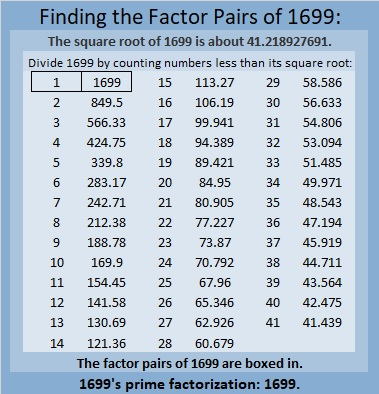How do we know that 1699 is a prime number? If 1699 were not a prime number, then it would be divisible by at least one prime number less than or equal to √1699. Since 1699 cannot be divided evenly by 2, 3, 5, 7, 11, 13, 17, 19, 23, 29, 31, 37, or 41, we know that 1699 is a prime number.

### More About the Number 1699:

1699 is the third prime in a prime triple. What are the other two primes in that triple?

1699 is the difference of two squares:
850² – 849² = 1699.

# 1697 A Boot in the Window

### Today’s Puzzle:

Tonight throughout many parts of the world children will place their polished boots in a window awaiting a visit from St. Nick. In the morning they will find their boots filled with favorite candies.

You can solve this boot puzzle by writing the numbers from 1 to 12 in both the first column and the top row so that those numbers and the given clues will become the start of a multiplication table.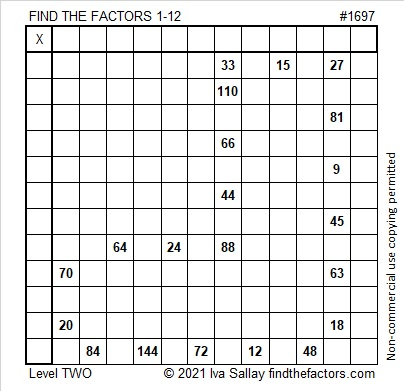### Factors of 1697:

• 1697 is a prime number.
• Prime factorization: 1697 is prime.
• 1697 has no exponents greater than 1 in its prime factorization, so √1697 cannot be simplified.
• The exponent in the prime factorization is 1. Adding one to that exponent we get (1 + 1) = 2. Therefore 1697 has exactly 2 factors.
• The factors of 1697 are outlined with their factor pair partners in the graphic below.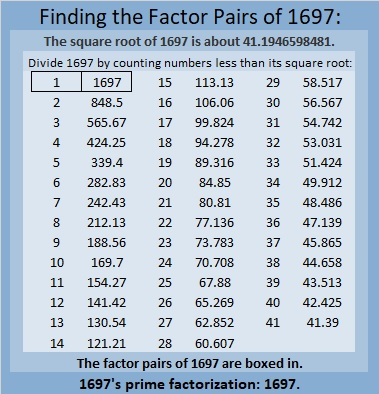How do we know that 1697 is a prime number? If 1697 were not a prime number, then it would be divisible by at least one prime number less than or equal to √1697. Since 1697 cannot be divided evenly by 2, 3, 5, 7, 11, 13, 17, 19, 23, 29, 31, 37, or 41, we know that 1697 is a prime number.

### More About the Number 1697:

1697 is the sum of two squares:
41² + 4² = 1697.

1697 is the hypotenuse of a Pythagorean triple:
328-1665-1697, calculated from 2(41)(4), 41² – 4², 41² + 4².

Here’s another way we know that 1697 is a prime number: Since its last two digits divided by 4 leave a remainder of 1, and 41² + 4² = 1697 with 41 and 4 having no common prime factors, 1697 will be prime unless it is divisible by a prime number Pythagorean triple hypotenuse less than or equal to √1697. Since 1697 is not divisible by 5, 13, 17, 29, 37, or 41, we know that 1697 is a prime number.

# 1693 Tricky Turkey

### Today’s Puzzle:

You cannot gobble this turkey up unless you can find all of its factors!

Use logic and multiplication facts. It won’t be easy, but write the numbers from 1 to 10 in both the first column and the top row so that those numbers and the given clues make a multiplication table.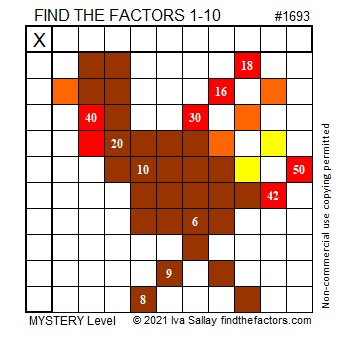Here’s the same puzzle without the added color:### Factors of 1693:

• 1693 is a prime number.
• Prime factorization: 1693 is prime.
• 1693 has no exponents greater than 1 in its prime factorization, so √1693 cannot be simplified.
• The exponent in the prime factorization is 1. Adding one to that exponent we get (1 + 1) = 2. Therefore 1693 has exactly 2 factors.
• The factors of 1693 are outlined with their factor pair partners in the graphic below.

How do we know that 1693 is a prime number? If 1693 were not a prime number, then it would be divisible by at least one prime number less than or equal to √1693. Since 1693 cannot be divided evenly by 2, 3, 5, 7, 11, 13, 17, 19, 23, 29, 31, 37, or 41, we know that 1693 is a prime number.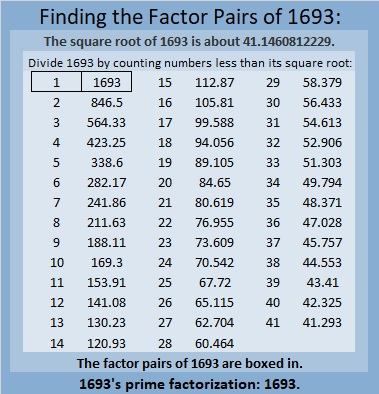### More About the Number 1693:

1693 is the sum of two squares:
37² + 18² = 1693.

1693 is the hypotenuse of a Pythagorean triple:
1045-1332-1693, calculated from 37² – 18², 2(37)(18), 37² + 18².

Here’s another way we know that 1693 is a prime number: Since its last two digits divided by 4 leave a remainder of 1, and 37² + 18² = 1693 with 37 and 18 having no common prime factors, 1693 will be prime unless it is divisible by a prime number Pythagorean triple hypotenuse less than or equal to √1693. Since 1693 is not divisible by 5, 13, 17, 29, 37, or 41, we know that 1693 is a prime number.

# 1669 and Level 6

### Today’s Puzzle:

Some sets of clues in this puzzle have two possible common factors, and another set has three possible common factors. Don’t guess which ones to use, but use logic instead! Write the numbers 1 to 12 in both the first column and the top row so that those numbers and the given clues form a multiplication table.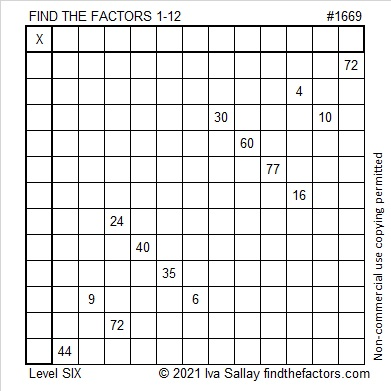### Factors of 1669:

• 1669 is a prime number.
• Prime factorization: 1669 is prime.
• 1669 has no exponents greater than 1 in its prime factorization, so √1669 cannot be simplified.
• The exponent in the prime factorization is 1. Adding one to that exponent we get (1 + 1) = 2. Therefore 1669 has exactly 2 factors.
• The factors of 1669 are outlined with their factor pair partners in the graphic below.How do we know that 1669 is a prime number? If 1669 were not a prime number, then it would be divisible by at least one prime number less than or equal to √1669. Since 1669 cannot be divided evenly by 2, 3, 5, 7, 11, 13, 17, 19, 23, 29, 31, or 37, we know that 1669 is a prime number.

### More About the Number 1669:

1669 is the sum of two squares:
38² + 15² = 1669.

1669 is the hypotenuse of a primitive Pythagorean triple:
1140-1219-1669, calculated from 2(38)(15), 38² – 15², 38² + 15².

Here’s another way we know that 1669 is a prime number: Since its last two digits divided by 4 leave a remainder of 1, and 38² + 15² = 1669 with 38 and 15 having no common prime factors, 1669 will be prime unless it is divisible by a prime number Pythagorean triple hypotenuse less than or equal to √1669. Since 1669 is not divisible by 5, 13, 17, 29, or 37, we know that 1669 is a prime number.